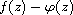Electron. J. Diff. Equ., Vol. 2014 (2014), No. 204, pp. 1-12.

### Growth of solutions of linear differential equations with analytic coefficients of [p,q]-order in the unit disc Hui Hu, Xiu-Min Zheng

Abstract:
In this article, we study the growth of solutions of homogeneous linear differential equation in which the coefficients are analytic functions of [p,q]-order in the unit disc. We obtain results about the (lower) [p,q]-order of the solutions, and the (lower) [p,q]-convergence exponent for the sequence of distinct zeros of.

Submitted October 23, 2013. Published September 30, 2014.
Math Subject Classifications: 30D35, 34M10.
Key Words: Unit disc; analytic function; (lower) [p,q]-order; (lower) [p,q]-type; (lower) [p,q]-convergence exponent.

Show me the PDF file (248 KB), TEX file, and other files for this article.Hui Hu Institute of Mathematics and Information Science Jiangxi Normal University, 330022, China email: h_h87_6@hotmail.com Xiu-Min Zheng Institute of Mathematics and Information Science Jiangxi Normal University, 330022, China email: zhengxiumin2008@sina.com